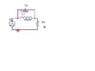# Laplace Step Response Circuit Analysis

• Engineering

## Homework Statement

Shown in attachment

## The Attempt at a Solution

I'm trying to analyze the circuit in the attached picture. This is a step response with a 3V input or 3u(t).

What I've done so far is:

1. convert all of the components to the s-domain.

R = R, L = sL, C = 1/sC

2. Combine the L and C into one impedence

[sL*(1/sC)]/[sL + (1/sC)] = Z

$\frac{7.4999999999999985\,{10}^{7}}{0.15\,s+\frac{4.9999999999999994\,{10}^{8}}{s}}$

3. Using voltage divider formula, solve for the voltage across R

Vout = (R*Vin)/(R+Z)

Vout = [R*(3/s)]/(R+Z)

4. Simplified all calculations

Vout = (3R/s)/R+Z

$\frac{9000.0}{\left( \frac{7.4999999999999985\,{10}^{7}}{0.15\,s+\frac{4.9999999999999994\,{10}^{8}}{s}}+3000.0\right) \,s}$

5. Evaluated inverse laplace transform of Vout using Maxima

$3-\frac{30\,{e}^{-\frac{250000\,t}{3}}\,\mathrm{sinh}\left( \frac{50000\,\sqrt{13}\,t}{3}\right) }{\sqrt{13}}$

After plugging in certain times and comparing them to actual measured values this circuit provides at the same times, I get step responses with percent differences ranging from 4-30%.

I feel like this is wrong since most formulas I've derived for earlier types of circuits resemble the real life results quite closely.

#### Attachments

•untitled.JPG
5.6 KB · Views: 426

## Homework Statement

Shown in attachment

## The Attempt at a Solution

I'm trying to analyze the circuit in the attached picture. This is a step response with a 3V input or 3u(t).

What I've done so far is:

1. convert all of the components to the s-domain.

R = R, L = sL, C = 1/sC

2. Combine the L and C into one impedence

[sL*(1/sC)]/[sL + (1/sC)] = Z

$\frac{7.4999999999999985\,{10}^{7}}{0.15\,s+\frac{4.9999999999999994\,{10}^{8}}{s}}$

3. Using voltage divider formula, solve for the voltage across R

Vout = (R*Vin)/(R+Z)

Vout = [R*(3/s)]/(R+Z)

4. Simplified all calculations

Vout = (3R/s)/R+Z

$\frac{9000.0}{\left( \frac{7.4999999999999985\,{10}^{7}}{0.15\,s+\frac{4.9999999999999994\,{10}^{8}}{s}}+3000.0\right) \,s}$

5. Evaluated inverse laplace transform of Vout using Maxima

$3-\frac{30\,{e}^{-\frac{250000\,t}{3}}\,\mathrm{sinh}\left( \frac{50000\,\sqrt{13}\,t}{3}\right) }{\sqrt{13}}$

After plugging in certain times and comparing them to actual measured values this circuit provides at the same times, I get step responses with percent differences ranging from 4-30%.

I feel like this is wrong since most formulas I've derived for earlier types of circuits resemble the real life results quite closely.

Can you fix your LaTex? It's hard to follow what you've done with the equations so battered lookingEven so, It doesn't appear to me that your resulting expression for the parallel impedance of the cap and coil looks right. C1 is 0.002 μF, right?

As gneill says, hard if not impossible to check the details.

As far as corroborating your empirical data with your analysis I would point out that, while the capacitor probably acts very close to an ideal capacitor, such is not true of the inductor, which has very appreciable equivalent resistance in series with it. Actually, that resistance is a function of frequency, and since your step input spans a large frequency range (|1/jw|) it is wishful thinking for you to hope that you will get the exact results you expect.

As an example: set your input sine generator to about 9.2 KHz. You should see a dip in the output around that frequency. The fact that you can't attain zero output is ascribable almost entirely to the finite "Q" of the inductor.

P.S. also vary the input voltage to make sure you're not saturating the inductor which at that value most probably has a high-permeability core.

Also following along the lines of what Rude Man has stated about the inductor, capacitors can have a rather large tolerance variations in their values. Some inexpensive capacitors can have values that stray -20% to +80% of their marked values. You may be able to locate a Tolerance Code on your capacitor to give you an idea of what range the actual capacitance will lie. Failing that, find a capacitance meter and measure it.

Also following along the lines of what Rude Man has stated about the inductor, capacitors can have a rather large tolerance variations in their values. Some inexpensive capacitors can have values that stray -20% to +80% of their marked values. You may be able to locate a Tolerance Code on your capacitor to give you an idea of what range the actual capacitance will lie. Failing that, find a capacitance meter and measure it.

Sure, but a 0.002uF capacitor is 2000 pF which will probably be a good-quality ceramic or something equally free from those problems. Totally ignorable compared to the inductor IMO.

Sure, but a 0.002uF capacitor is 2000 pF which will probably be a good-quality ceramic or something equally free from those problems. Totally ignorable compared to the inductor IMO.

True, it depends upon the type of capacitor. Still, there will be some variance from the stated value. For a lab where one should do error analysis, it would make sense to check.

I would think that an inductor as large as 150 mH would have a DC resistance on the order of 200 to 300 Ohms.